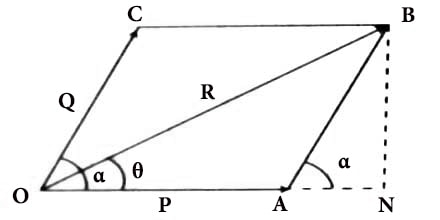Physics

# Minimum Value of ResultantSuppose two vectors P and Q are acting simultaneously at a point making an angle α. Now according to parallelogram law the magnitude of the resultant.

R = √(P2 + Q2 + 2PQ cosα)

From the above equation it is evident that R depends on the angle between P and Q i.e., on α.Minimum value of the resultant: The magnitude of R will be minimum when cos α is minimum i.e., cos α = – 1 = cos 180° or, α = 180°.

R(minimum) = √(P2 + Q2 + 2PQ cos 1800)

= √(P2 + Q2 ~ 2PQ)

= (P ~ Q)

Thus, when two vectors act along the same line but in opposite direction, the magnitude of the resultant will be minimum. In other words, the minimum value of magnitude of the resultant of the two vectors cannot be less than their subtraction.

(Here, ~ sign between P and Q means that the larger one should be written first e.g., suppose Q > P, then P ~ Q = Q – P)

If two equal and opposite forces act along the ends of a straight line, the resultant will be zero.